Next: Kepler's First Law Up: Planetary Motion Previous: Conic Sections

# Kepler's Second Law

Multiplying our planet's tangential equation of motion, (229), by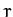, we obtain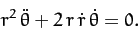(245)

However, the above equation can be also written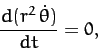(246)

which implies that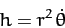(247)

is constant in time. It is easily demonstrated that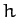is the magnitude of the vector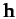defined in Equation (216). Thus, the fact thatis constant in time is equivalent to the statement that the angular momentum of our planet is a constant of its motion. As we have already mentioned, this is the case because gravity is a central force.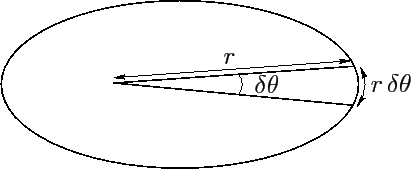Suppose that the radius vector connecting our planet to the origin (i.e., the Sun) sweeps out an angle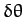between times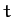and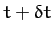--see Figure 18. The approximately triangular region swept out by the radius vector has the area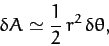(248)

since the area of a triangle is half its base (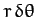) times its height (). Hence, the rate at which the radius vector sweeps out area is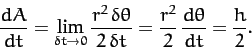(249)

Thus, the radius vector sweeps out area at a constant rate (sinceis constant in time)--this is Kepler's second law. We conclude that Kepler's second law of planetary motion is a direct consequence of angular momentum conservation.Next: Kepler's First Law Up: Planetary Motion Previous: Conic Sections
Richard Fitzpatrick 2011-03-31